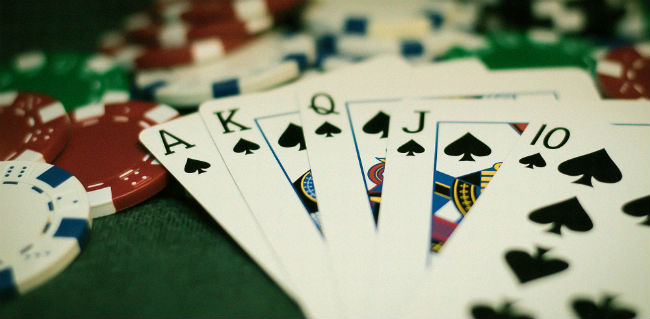# Poker probabilitiesPoker is a game about probability. It does not matter how good you are at reading people, if you do not understand the maths that underpin this great game then you are going to lose.

## What is Probability?

To put it simply, probability is the likelihood of a particular outcome occurring. For example, when you two a coin there are two possible results. The probability of one of these results occurring is 50% or one in two.

## Probability in Card Games

Unlike a coin, there are many different outcomes when dealing a deck of cards. A deck of cards contains 52 unique cards spread across 4 suits and thirteen ranks. So the probability of the top card being the Ace of Hearts is 1.9% (1/52), while the probability of it being any of the Aces is 7.7% (1/13).

Unlike with coins, the previous cards dealt affect the likelihood of the next cards occurring. For example, if the first card is a King of Clubs then the odds that the next card is a King is now 5.9% (3/51) while the odds that it is a Queen is 7.8% (4/51).

## The Probability of a Pair Pre-Flop

To find the likelihood of getting a particular pair, simply multiply the probabilities of each card together. So the odds of starting with a pair of Jacks is:

7.7% (4/52) x 5.9% (3/51) = 0.45%

This means that it would take you 7.5 hours to see a particular pair pre-flop if you were to be dealt 30 hands every hour.

However, if you want to receive any pocket pair pre-flop your odds are 5.9% (3/51). At the same rate of 30 hands each hour, it should take 35 minutes to be dealt any pair.

## Probabilities of Winning Pre-Flop

Since poker is not a single player game, you have to account for the probability of what an opponent may have. To give a quick overview, if you have a high-pair pre-flop then you have about an 82% chance of winning. If you have two high cards, then your chance of beating an opponent, even one with a low pair which beats you for the moment, is still 55%.

The pre-flop is only the beginning of the game. What matters is how likely your hand is to get better after the flop. Here are some odds:

• Non-pairs have a 32% chance to pair.
• Two suited cards have a 6.5% chance to make a flush.
• Two suited cards have a 0.85% chance to flop a flush.
• Two suited cards have a 10.9% chance to flop a four flush.
• A pair has a 12% chance to flop a set.
• A pair has a 0.25% chance to flop as a four-of-a-kind.

So be wary of overvaluing particular starting hands such as suited cards and small pairs.

## Playing to Outs

Knowing the odds of your or your opponents’ hand improving is important. An “out” is any card that will improve a hand.

Trying to calculate all the outs can be difficult but there is a useful shortcut the “rule of four and two”. First, count the number of cards that will improve your hand and multiply that by 4. This gives you the odds of improving your hand on the turn. If you miss the draw on the river’s turn then you multiply your outs by 2 instead.

## Pot Odds

Pot odds take the size of the pot and judge the proportion of the next bet against it. For example, if the current pot is £90 and a £10 bet is required to stay in, then this is called 9 to 1 pot odds. Then £10 bet will be making up 10% of the new pot.

You can utilise this by comparing the pot odds to the chances of your hand improving. If your chances for improving your hand are better than the pot odds, call the bet. If they are not then you should fold.